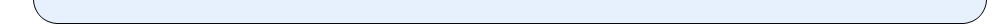••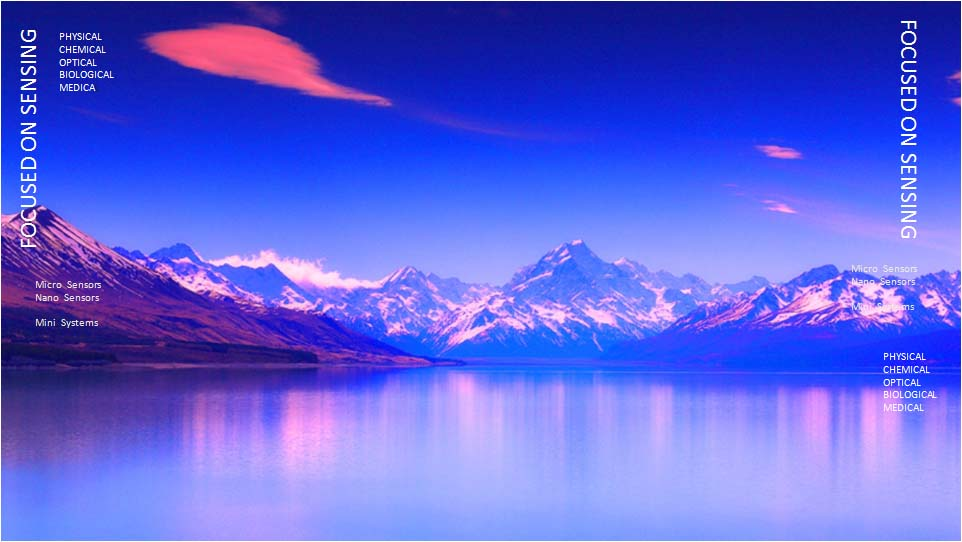•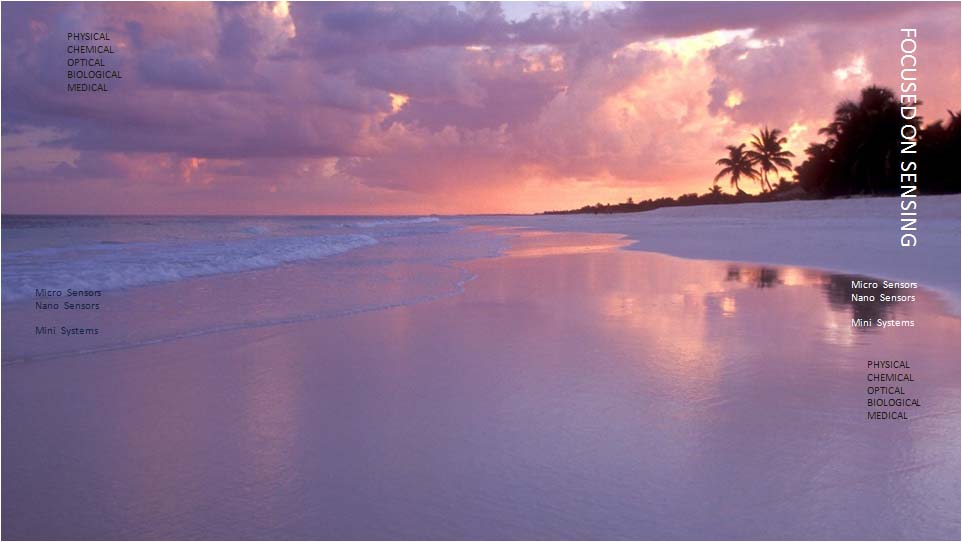•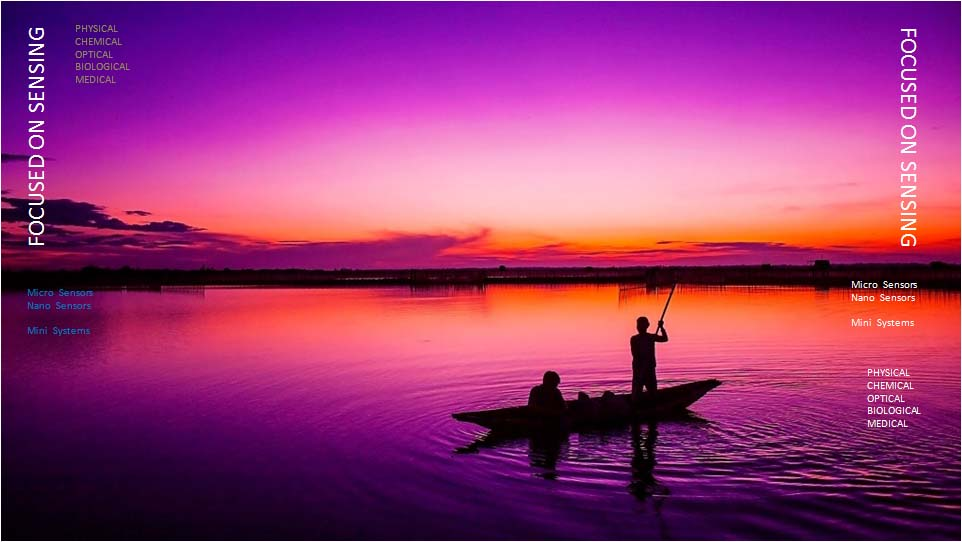•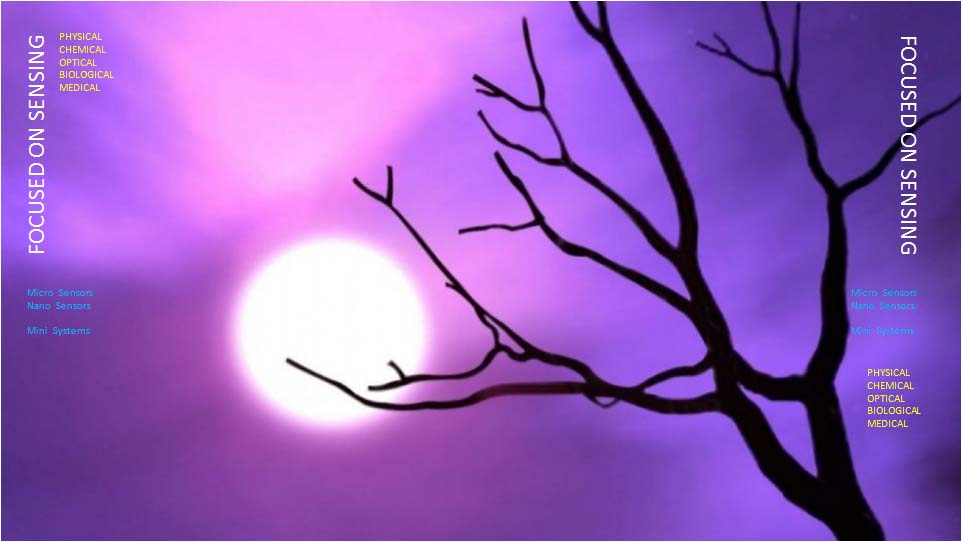•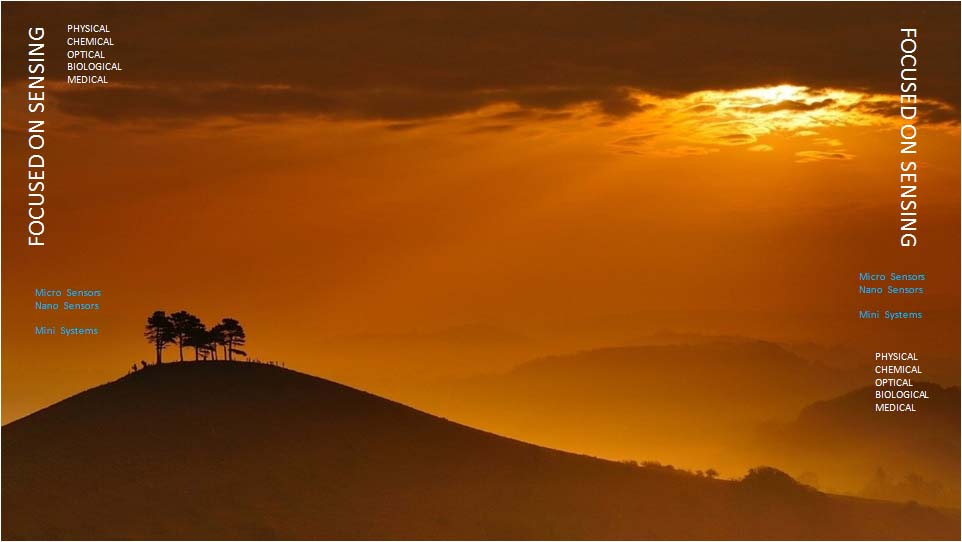•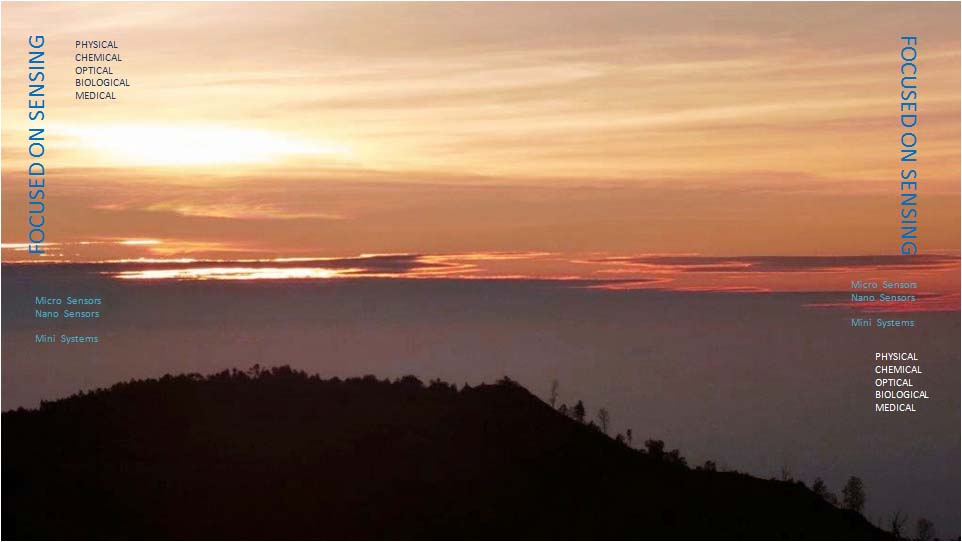•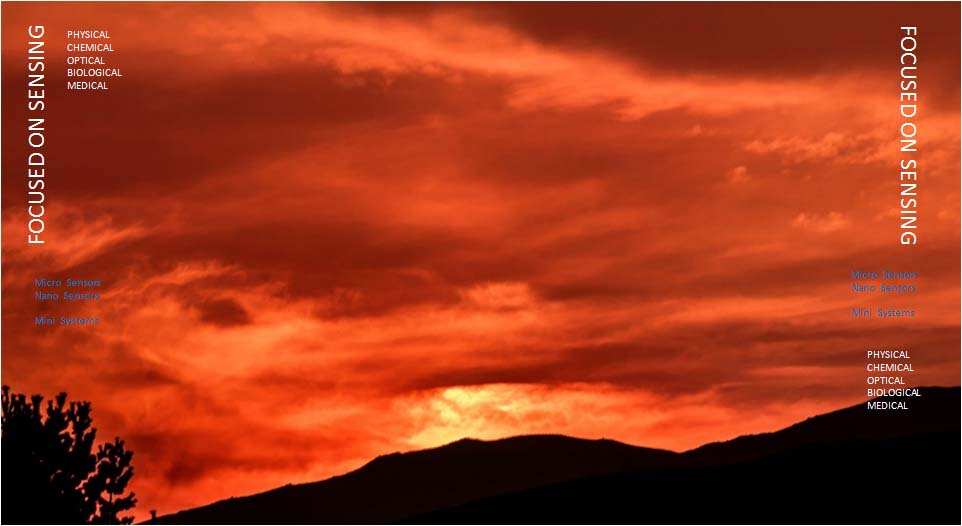•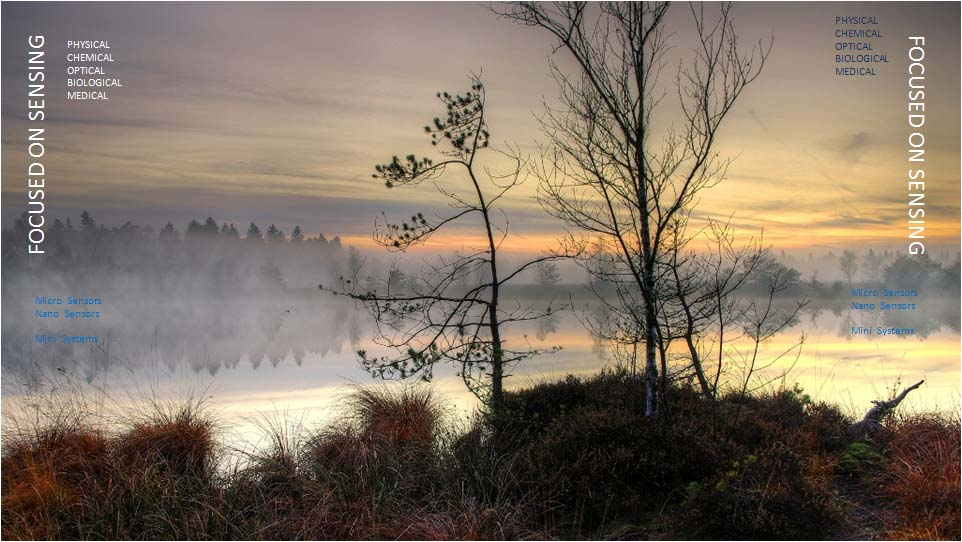•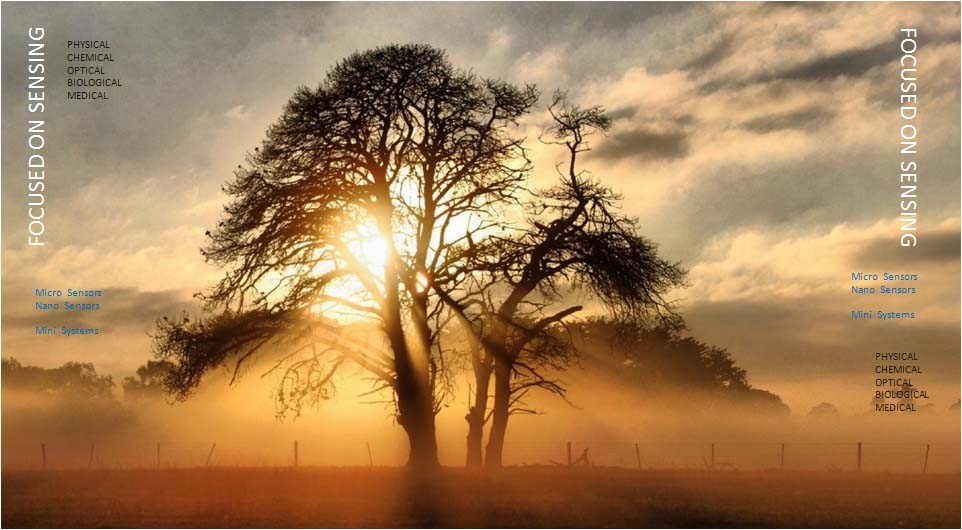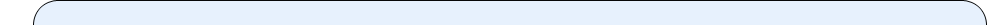|   CONCEPT   ANALYSIS  |

While developing a sensor, initially a concept is developed for the measurand. Then, the sensing concept is analyzed using simplified mathematical tools. Simple closed form formulation are used to check out the feasibility of the concept. For example, to measure a pressure change, a diaphragm of proper thickness (plate) can be used which deflects due to the smallest change in pressure to be measured. The deflection of the diaphragm can be measured directly using reflected optics or indirectly from the stresses developed at the supporting perimeter of the diaphragm.

As the stresses can be determined from the strain developed at the stressed location; one builds strategically diffused strain gages to indirectly measure the pressure change on a diaphragm. Most of the silicon pressure sensors manufactured today use this concept. The pressure transducer included in ANDAS is made using this concept.

Examples of other physical sensing concept include:

• Accelerometer: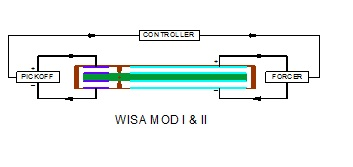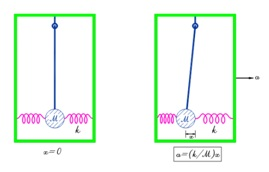It is built as a device where a mass is supported by a weak spring in a box. When the box is subjected to an acceleration, the mass inside tends to stay inertial; and by measuring the relative displacement between the mass and the box, through spring constant one measures the restoring force, which when divided by the mass yields the acceleration (Newton’s laws used for an Open Loop Accelerometer concept shown in the figure above on the left). For better accuracy, an electrical rebalancing loop is employed in closed loop accelerometers  as shown in figure on the right,
• Gyros: When a rotary wheel having constant angular momentum is subjected to an angular rotation perpendicular to the angular momentum, the rotary wheel precesses about an axis perpendicular to the applied angular rotation, (RG)
• Stress: By embedding a strain rosette at the stressed location, (ANDAS)
• Temperature: By embedding diffused resistor or a diode highly sensitive to temperature, one can measure the temperature, (ANDAS)
• Sound Level: By embedding microphone or a hydrophone, which is essentially a differential pressure transducer.

Examples of other optical sensing concept include:

• Laser Gyro (or Fiber Optic Gyro): If a planar ring structure has a fixed location where two counter propagating beams start, they will come back to the starting point at the same time if the structure is stationary. If the structure is rotated in its plane, the beam that has to travel longer distance will arrive later,
• Accelerometer: Acceleration applied to a structure generate inertial load in the structure; which in turn affects the speed of light propagation,
• Laser Based Gas Sensing: The absorption of light passing through a gaseous medium depends upon the wavelength of light.

Examples of other Fluidic Sensing Concept include:

• Fluidic Metering: Fluidic amplifier affected by vacuum lines controls fuel flow to an internal combustion engine, thus, effectively behaving like a carburetor,
• Gyro and Accelerometer: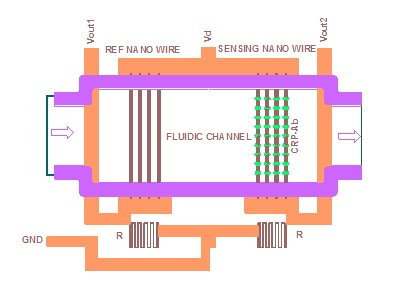The amplification produced by a fluidic amplifier is proportional to lateral inertial forces applied.
• Viscosity: Under a fixed pressure, the flow speed of a fluid through a fluidic path depends on its viscosity
• Flow across SiC Nanowires: Nanowires with self-assembled sensing molecules placed in one leg of a measurement bridge can be used for water toxicity measurement, blood testing, and measurement of other body fluids as biomarkers as shown in the figure on the right.

Example of other Chemical Sensing Concept:

• Gas Sensing: The resistivity of some heated metallic films changes in the presence of a specific gas.
•